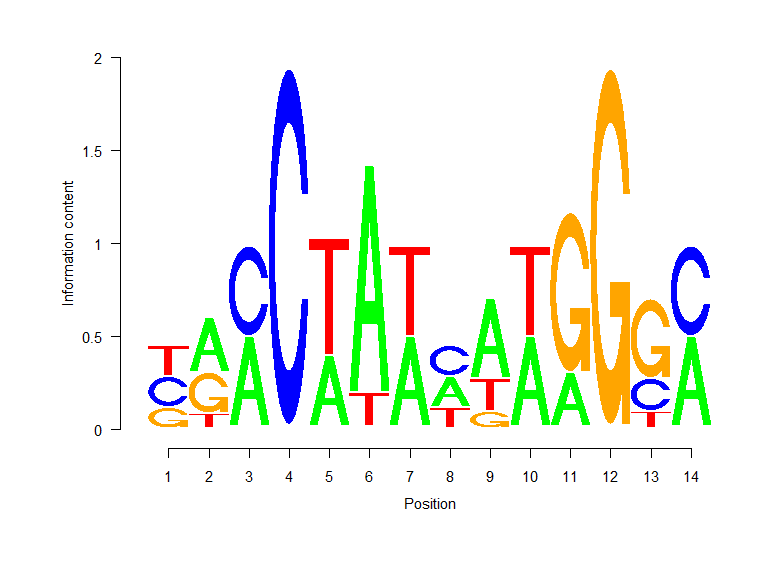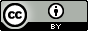# Position weight matrix

The process of transcription, is influenced by the interaction of proteins called transcription factors (TFs) that bind to specific sites called Transcription Factor Binding Sites (TFBSs), which are proximal or distal to a transcription starting site. TFs generally have distinct binding preferences towards specific TFBSs, however TFs can tolerate variations in the target TFBS. Thus to model a TFBS, the nucleotides are weighted accordingly, to the tolerance of the TF. One common way to represent this is by using a position weight matrix (PWM), also called position-specific weight matrix (PSWM) or position-specific scoring matrix (PSSM), which is a commonly used representation of motifs (in our case TFBS) in biological sequences.

How do we find TFBSs? DNA sequences that interact with TFs can be experimentally determined from SELEX experiments. Since this process involves synthesis of a large number of randomly generated oligonucleotides, DNA sequences that interact with TFs can be determined, as well as the tolerance at specific sites. From SELEX experiments, a position frequency matrix (PFM) can be constructed by recording the position-dependent frequency of each nucleotide in the DNA sequence that interacted with the TF. Here’s an example of a PFM as shown in this review “Applied bioinformatics for the identification of regulatory elements” (sorry paywall!):

 1 2 3 4 5 6 7 8 9 10 11 12 13 14 A 0 4 4 0 3 7 4 3 5 4 2 0 0 4 C 3 0 4 8 0 0 0 3 0 0 0 0 2 4 G 2 3 0 0 0 0 0 0 1 0 6 8 5 0 T 3 1 0 0 5 1 4 2 2 4 0 0 1 0

The construction of this PFM was done by collecting experimentally validated binding sites from 8 published studies for MEF2.

To convert a PFM to the corresponding PWM, the frequencies are converted to normalised frequency values on a log-scale. To perform this conversion we can use these formulae from the review paper:$W_{b,i} = log_{2}\frac{p(b,i)}{p(b)}$

where$W_{b,i}$ = PWM value of base b in position i,$p(b)$ = background probability of base b and$p(b,i)$:$p(b,i) = \frac{f_{b,i} + s(b)}{N + \sum s(b')}$

where$b' \in \{A, C, G, T\}$;$f_{b,i}$ = counts of base b in position i;$N$ = number of sites;$p(b,i)$ = corrected probability of base b in position i and$s(b)$ = pseudocount function.

The pseudocount is a sample correction that is added when assessing the probability to correct for small samples sizes and this calculation varies widely between applications. One approach is to take the square root of the number of sites that contribute to the model, which was apparently the approach used in the paper. However, when I used this as the pseudocount function, I could not replicate the numbers in the table of the review (Box 1, d). In fact, if you examine the PWM in the paper (Box 1, d), there’s several typos. For example, in position 1, the frequency of C’s and T’s are identical, however the PWM values are different. Also the PWM for a frequency of 1 is different in position 13, T and all other positions with a frequency of 1. Most other values seem to be consistent though. So in order to find out the pseudocount function, I substituted some of the (consistent) PWM values back into the equation.

Firstly recall the conversion between the logarithmic form to the exponential form:$y = log_{a}x \leftrightarrow a^{y} = x.$

Therefore:$W_{b,i} = log_{2}\frac{p(b,i)}{p(b)} \leftrightarrow 2^{W_{b,i}} = \frac{p(b,i)}{p(b)}$

and substituting$p(b,i)$ in:$2^{W_{b,i}} \cdot p(b) = \frac{f_{b,i} + s(b)}{N + \sum s(b')}$

The PWM value for a frequency of 0 seems to be consistent, so let’s take$W_{A,1}$ and substitute it into the equation:$2^{-1.93} \cdot p(b) = \frac{0 + s_{A}}{8 + s_{A} + s_{C} + s_{G} + s_{T}}.$

The PWM value for a frequency of 3 seems to be 0.45, so let’s take$W_{C,1}$ and substitute it into the equation:$2^{0.45} \cdot p(b) = \frac{3 + s_{C}}{8 + s_{A} + s_{C} + s_{G} + s_{T}}.$

We now have two simultaneous equations that we can divide together, i.e. dividing$W_{A,1}$ by$W_{C,1}$ (the denominators cancel each other out), to work out the pseudocount:$\frac{2^{-1.93} \cdot p(b)}{2^{0.45} \cdot p(b)} = \frac{s_{A}}{3 + s_{C}}.$

The background probabilities cancel each other out and since the pseudocounts should be the same for the different nucleotides, we can refer to them as an s:$0.1921094 = \frac{s}{3 + s}.$

Invert the two sides:$5.205367 = \frac{3 + s}{s} = \frac{3}{s} + \frac{s}{s}.$

Solving s, we get:$5.205367 = \frac{3}{s} + 1$$4.205367 = \frac{3}{s}$$s = \frac{3}{4.205367} = 0.7133741$

Perhaps I missed it, but it wasn’t pointed out exactly how$p(b)$ or the background probability of base b is defined. Since we worked out s, we can substitute it into the equation for$W_{A,1}$ and work out$p(b)$:$2^{-1.93} \cdot p(b) = \frac{0 + 0.71}{8 + 0.71 + 0.71 + 0.71 + 0.71}.$$p(b) = \frac{0.06572758}{2^{-1.93}} = 0.2504584.$

So the background probability of base b is simply 0.25, i.e. base b divided by the total number of bases. But where did this 0.71 pseudocount come from? If we take the square root of the number of sites that contribute to the model, the square root of 8 is 2.828427. Perhaps the pseudocount needs to be scaled by base b, since the square root of 8 multiply by 1/4 is 0.7071068.

Since some of the values in the PWM of the review paper is incorrect, let’s calculate it again using the formulae above (since we now know the pseudocount function and the background probability) using R:

#function for working out the position weight matrix value
pwm <- function(freq, total, bg=0.25){
#using the formulae above
p <- (freq + (sqrt(total) * 1/4)) / (total + (4 * (sqrt(total) * 1/4)))
log2(p/bg)
}
#work out all possible PWM values
for (i in 0:8){
print(pwm(i,8))
}
 -1.936752
 -0.6651985
 0
 0.4535419
 0.7980888
 1.076008
 1.308939
 1.509438
 1.685442


Now let’s calculate the PWM:

#define the frequencies of nucleotides
a <- c(0, 4, 4, 0, 3, 7, 4, 3, 5, 4, 2, 0, 0, 4)
c <- c(3, 0, 4, 8, 0, 0, 0, 3, 0, 0, 0, 0, 2, 4)
g <- c(2, 3, 0, 0, 0, 0, 0, 0, 1, 0, 6, 8, 5, 0)
t <- c(3, 1, 0, 0, 5, 1, 4, 2, 2, 4, 0, 0, 1, 0)
m <- matrix(data=c(a,c,g,t),nrow=4,byrow=T,dimnames=list(c('a','c','g','t')))
m
[,1] [,2] [,3] [,4] [,5] [,6] [,7] [,8] [,9] [,10] [,11] [,12] [,13] [,14]
a    0    4    4    0    3    7    4    3    5     4     2     0     0     4
c    3    0    4    8    0    0    0    3    0     0     0     0     2     4
g    2    3    0    0    0    0    0    0    1     0     6     8     5     0
t    3    1    0    0    5    1    4    2    2     4     0     0     1     0

mm <- pwm(m,8)
mm
[,1]       [,2]       [,3]      [,4]       [,5]       [,6]       [,7]       [,8]       [,9]
a -1.9367518  0.7980888  0.7980888 -1.936752  0.4535419  1.5094376  0.7980888  0.4535419  1.0760078
c  0.4535419 -1.9367518  0.7980888  1.685442 -1.9367518 -1.9367518 -1.9367518  0.4535419 -1.9367518
g  0.0000000  0.4535419 -1.9367518 -1.936752 -1.9367518 -1.9367518 -1.9367518 -1.9367518 -0.6651985
t  0.4535419 -0.6651985 -1.9367518 -1.936752  1.0760078 -0.6651985  0.7980888  0.0000000  0.0000000
[,10]     [,11]     [,12]      [,13]      [,14]
a  0.7980888  0.000000 -1.936752 -1.9367518  0.7980888
c -1.9367518 -1.936752 -1.936752  0.0000000  0.7980888
g -1.9367518  1.308939  1.685442  1.0760078 -1.9367518
t  0.7980888 -1.936752 -1.936752 -0.6651985 -1.9367518


Now that we have the PWM, we can generate a quantitative score for any DNA sequence (of the same length) by summing the values that correspond to the observed nucleotides at each position. Using R again:

#same sequence shown in the paper
seq <- 'ttacataagtagtc'
x <- strsplit(x=seq,split='')
x
[]
 "t" "t" "a" "c" "a" "t" "a" "a" "g" "t" "a" "g" "t" "c"
#initialise vector
seq_score <- vector()
#get the corresponding values
for (i in 1:14){
seq_score[i] <- mm[x[][i],i]
}
seq_score
  0.4535419 -0.6651985  0.7980888  1.6854416  0.4535419 -0.6651985  0.7980888  0.4535419 -0.6651985  0.7980888
  0.0000000  1.6854416 -0.6651985  0.7980888
#slightly different score to the paper due to their rounding
sum(seq_score)
 5.26307

#max score
sum(apply(mm,2,max))
 14.31481


Lastly, I’ve shown previously how to generate a sequence logo from a PFM, and following the same instructions:

#install if necessary
source("http://bioconductor.org/biocLite.R")
biocLite("seqLogo")

library(seqLogo)

a <- c(0, 4, 4, 0, 3, 7, 4, 3, 5, 4, 2, 0, 0, 4)
c <- c(3, 0, 4, 8, 0, 0, 0, 3, 0, 0, 0, 0, 2, 4)
g <- c(2, 3, 0, 0, 0, 0, 0, 0, 1, 0, 6, 8, 5, 0)
t <- c(3, 1, 0, 0, 5, 1, 4, 2, 2, 4, 0, 0, 1, 0)

df <- data.frame(a,c,g,t)
df
a c g t
1  0 3 2 3
2  4 0 3 1
3  4 4 0 0
4  0 8 0 0
5  3 0 0 5
6  7 0 0 1
7  4 0 0 4
8  3 3 0 2
9  5 0 1 2
10 4 0 0 4
11 2 0 6 0
12 0 0 8 0
13 0 2 5 1
14 4 4 0 0

#define function that divides the frequency by the row sum i.e. proportions
proportion <- function(x){
rs <- sum(x);
return(x / rs);
}

#create position weight matrix
mef2 <- apply(df, 1, proportion)
mef2 <- makePWM(mef2)
seqLogo(mef2)### Conclusions

With respect to transcription factors (TFs), a position weight matrix (PWM) can be generated from a position frequency matrix (PFM), which is a collection of experimentally validated binding sites. Using this PWM, any given sequence can be quantitatively scored against the motif model. The PWM models appropriately the tolerance of TFs to binding sites and one can use sequence logos to visualise PFMs.

I’d like to thank my colleague (if he ever comes across this page) for his help..
1.Abhi says:

Would be helpful to also have some explanation about information content in the PWM , as that’s what the logo is actually showing

2.Gwang-Jin says:

Dear Dave,
thank you for your excellent blogs!
Just a small typo: The equation after “There:” (beginning with W_{b, i}) the middle equation sign should be a doublearrow like in the equation before.

1.Davo says:

Thank you! I’ve made the amendment.

This site uses Akismet to reduce spam. Learn how your comment data is processed.